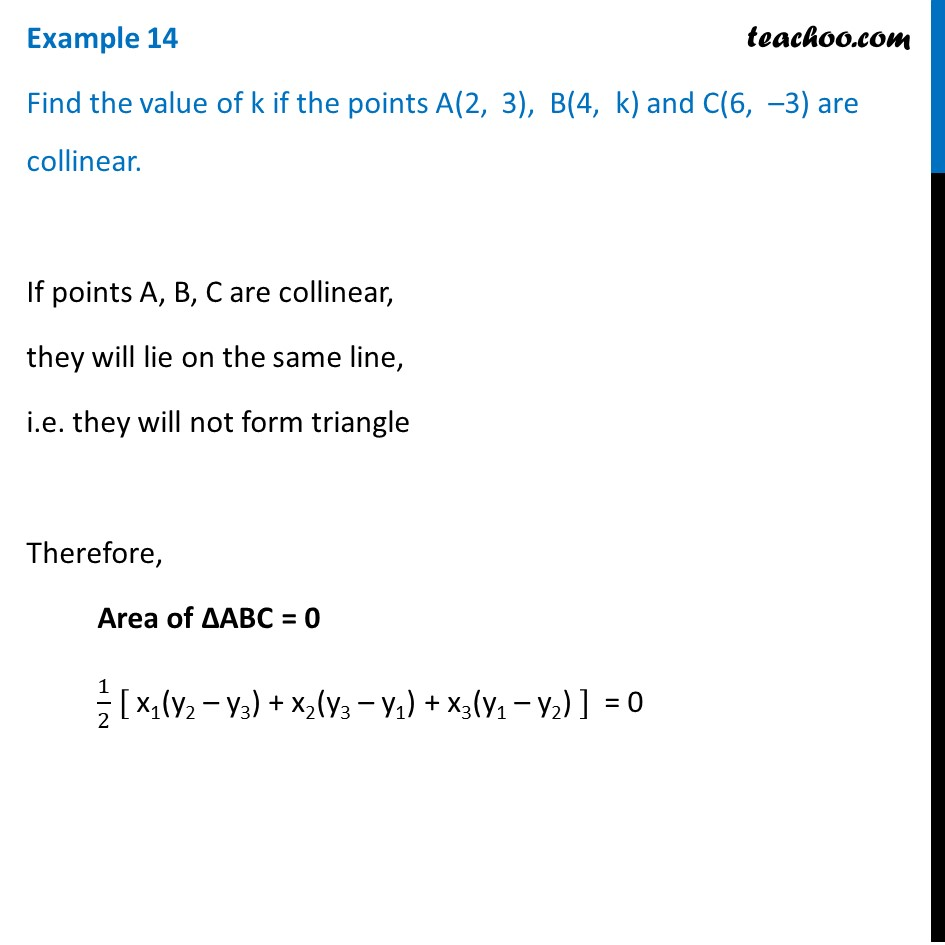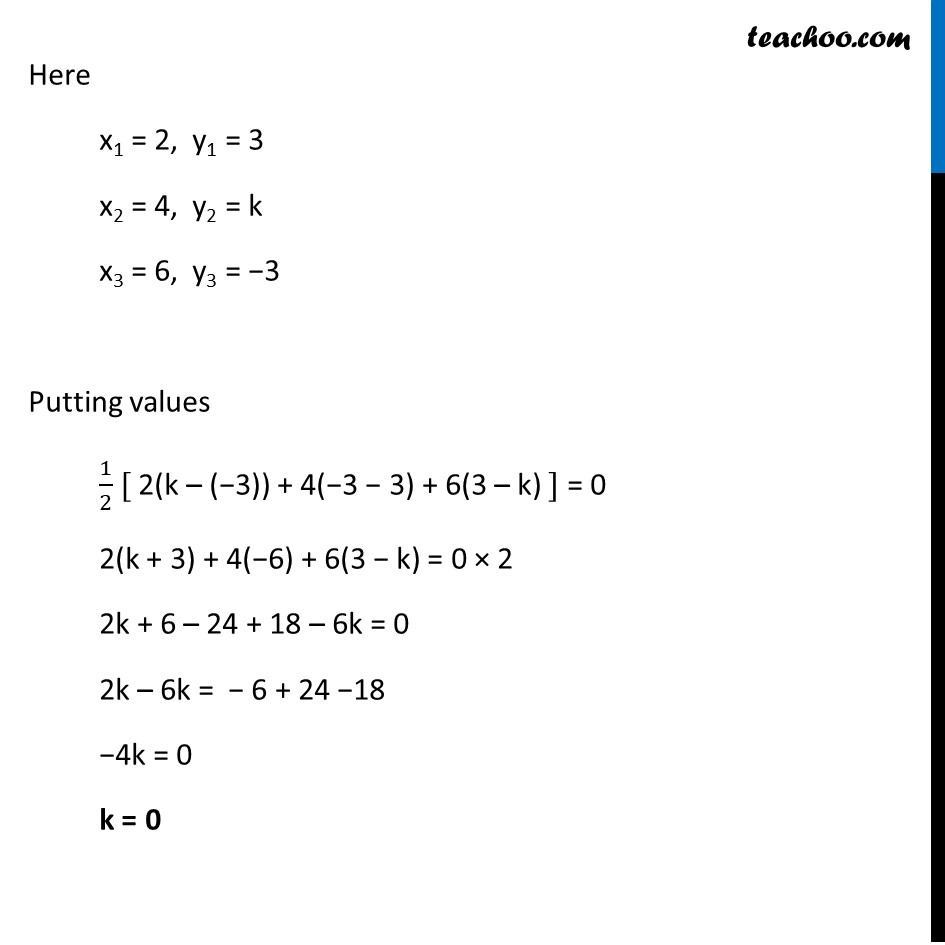Given area, finding k

Chapter 7 Class 10 Coordinate Geometry
Concept wiseLearn in your speed, with individual attention - Teachoo Maths 1-on-1 Class

### Transcript

Question 4 Find the value of k if the points A(2, 3), B(4, k) and C(6, –3) are collinear. If points A, B, C are collinear, they will lie on the same line, i.e. they will not form triangle Therefore, Area of ∆ABC = 0 1/2 [ x1(y2 – y3) + x2(y3 – y1) + x3(y1 – y2) ] = 0 Here x1 = 2, y1 = 3 x2 = 4, y2 = k x3 = 6, y3 = −3 Putting values 1/2 [ 2(k – (−3)) + 4(−3 − 3) + 6(3 – k) ] = 0 2(k + 3) + 4(−6) + 6(3 − k) = 0 × 2 2k + 6 – 24 + 18 – 6k = 0 2k – 6k = − 6 + 24 −18 −4k = 0 k = 0 Here x1 = 2, y1 = 3 x2 = 4, y2 = k x3 = 6, y3 = −3 Putting values 1/2 [ 2(k – (−3)) + 4(−3 − 3) + 6(3 – k) ] = 0 2(k + 3) + 4(−6) + 6(3 − k) = 0 × 2 2k + 6 – 24 + 18 – 6k = 0 2k – 6k = − 6 + 24 −18 −4k = 0 k = 0